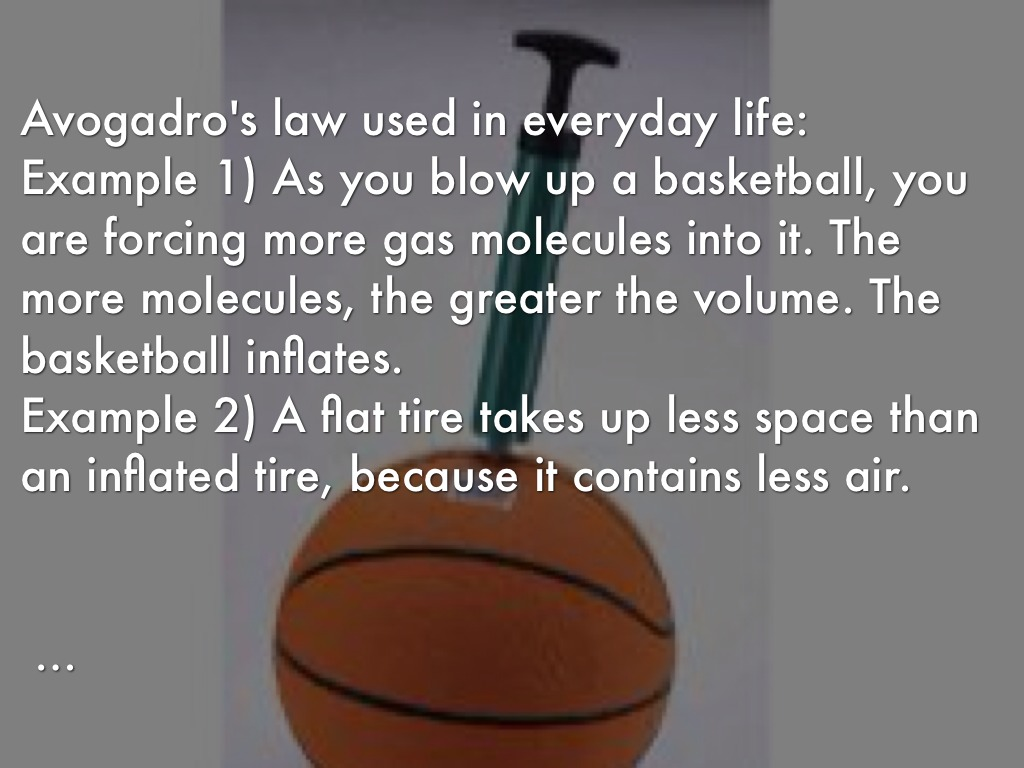## DescriptionAvogadro’s law is an experimental gas law relating volume of a gas to the amount of substance of gas present. Avogadro’s law states that, “equal volumes of all gases, at the same temperature and pressure, have the same number of molecules”.
For a given mass of an ideal gas, the volume and amount (moles) of the gas are directly proportional if the temperature and pressure are constant.
his law explains how, under the same condition of temperature and pressure, equal volumes of all gases contain the same number of molecules. For comparing the same substance under two different sets of conditions, the law can be also expressed as V1/V2=n1/n2.
The equation shows that, as the number of moles of gas increases, the volume of the gas also increases in proportion. Similarly, if the number of moles of gas is decreased, then the volume also decreases. Thus, the number of molecules or atoms in a specific volume of ideal gas is independent of their size or the molar mass of the gas.

Related formulas

## Variables

 V1 volume of the gas under conditions 1 (m3) n1 amount of the gas under conditions 1 (mol) V2 volume of the gas under conditions 2 (m3) n2 amount of the gas under conditions 2 (mol)